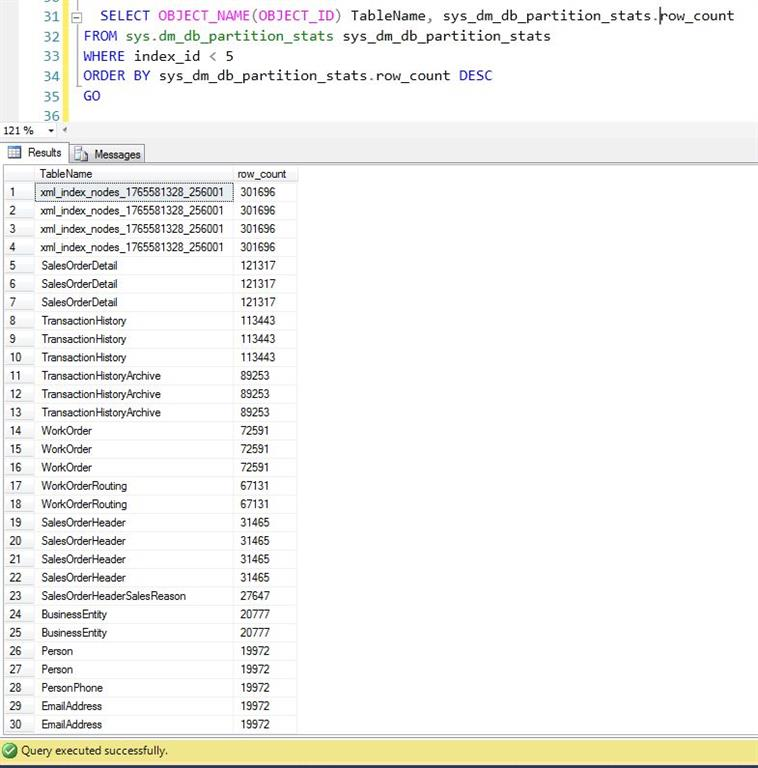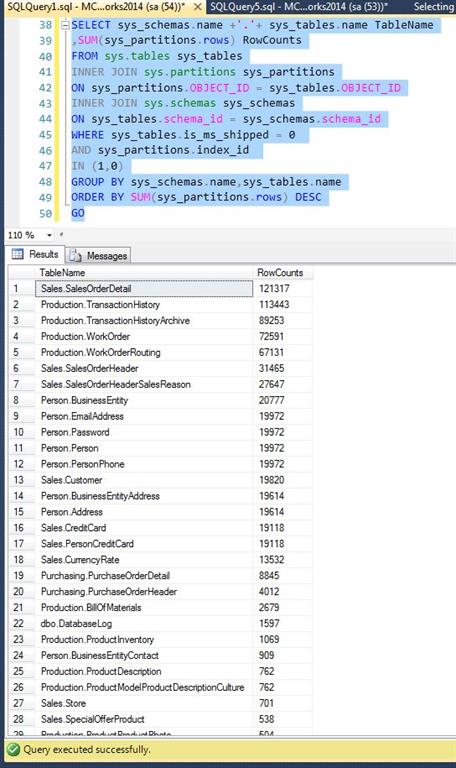In focus

# How To Count The Number Of Rows For User And System Tables Of A Database

## In this article, we will see how to countrows in table andthe largest row table in database in Transact-SQL..

Gagan Sharma Apr 20, 2016

In some cases our need is to find out which table consists of the maximum number of Rows or to find out the total number of rows in the database that includes both System and User tables. To find this out using Transact-SQL, follow the below given examples.

Example: We will find outthe  count of rows of tables inthe database that includes both User tables and System tables, In which RowsCounts is in descending order. We will use 'sys.dm_db_partition_stats'. There are 341 User and System tables in below given database.

Query:
1. SELECT OBJECT_NAME(OBJECT_ID) TableName, sys_dm_db_partition_stats.row_count
2. FROM sys.dm_db_partition_stats sys_dm_db_partition_stats
3. WHERE index_id < 5
4. ORDER BY sys_dm_db_partition_stats.row_count DESC
5. GO
Output:Example: Now in this example, We will find out count of rows of only User tables in database, in which RowsCounts is in descending order. We will use 'sys.partitions', 'sys.tables' and 'sys.schemas'. There are 71 User tables in my database.

Query:
1. SELECT sys_schemas.name +'.'+ sys_tables.name TableName
2. ,SUM(sys_partitions.rows) RowCounts
3. FROM sys.tables sys_tables
4. INNER JOIN sys.partitions sys_partitions
5. ON sys_partitions.OBJECT_ID = sys_tables.OBJECT_ID
6. INNER JOIN sys.schemas sys_schemas
7. ON sys_tables.schema_id = sys_schemas.schema_id
8. WHERE sys_tables.is_ms_shipped = 0
9. AND sys_partitions.index_id
10. IN (1,0)
11. GROUP BY sys_schemas.name,sys_tables.name
12. ORDER BY SUM(sys_partitions.rows) DESC
13. GO
Output: